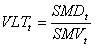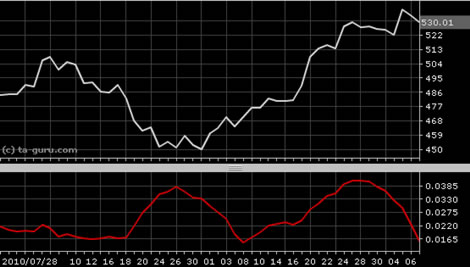Chart Pro online
User name (email):

 Recently used

 Forex

 Indices

# Volatility (VLT)

Volatility (VLT) is indicator in technical analysis which is statistical measure of volatility called coefficient of variation. It is calculated using two other indicators: standard deviation (SMD) and standard variance (SMV).

Volatility is calculated by formula:Default value for SMD and SMV period is 21.Volatility (VLT)

Interpretation

Volatility is not indicator which is used for creating buying and selling signals, it is more used as confirming indicator.

Example